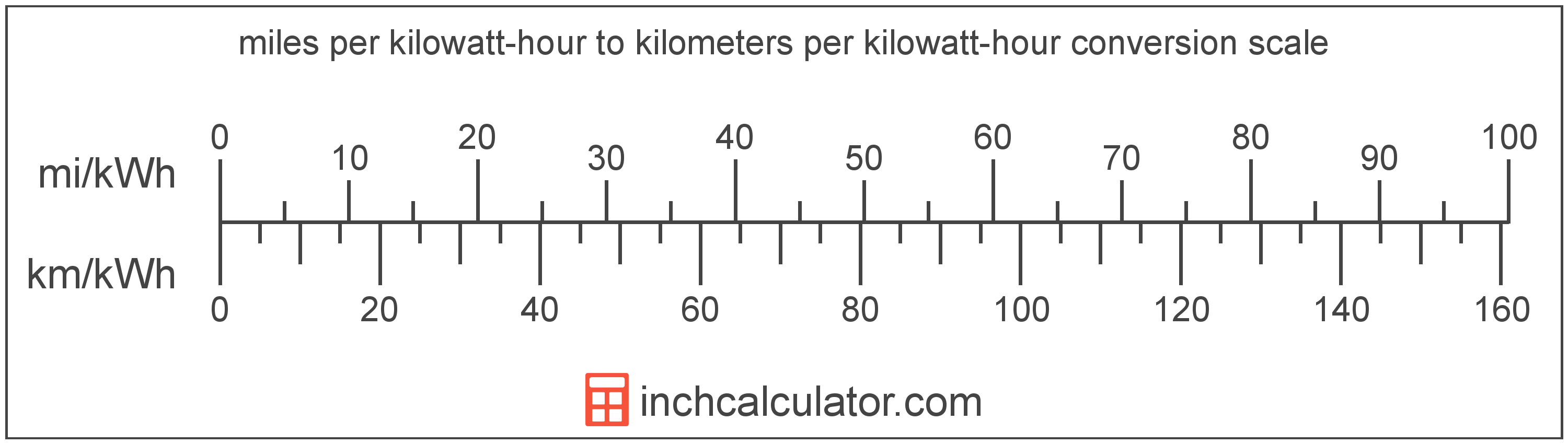# Miles per Kilowatt-Hour to Kilometers per Kilowatt-Hour Converter (Mi/kWh to Km/kWh)

Enter the electric car efficiency in miles per kilowatt-hour below to get the value converted to kilometers per kilowatt-hour.

Results in Kilometers per Kilowatt-Hour:1 mi/kWh = 1.609344 km/kWh

Do you want to convert km/kWh to mi/kWh?

## How to Convert Miles per Kilowatt-Hour to Kilometers per Kilowatt-Hour

Convert miles per kilowatt-hour to kilometers per kilowatt-hour with this simple formula:

km/kWh = mi/kWh × 1.609344

Insert the mi/kWh efficiency measurement in the formula and then solve to find the result.

For example, let's convert 50 mi/kWh to km/kWh:

50 mi/kWh = ( 50 × 1.609344 ) = 80.4672 km/kWhMiles per kilowatt-hour and kilometers per kilowatt-hour are both units used to measure electric car efficiency. Keep reading to learn more about each unit of measure.

## What Are Miles per Kilowatt-Hour?

Miles per kilowatt-hour is a measure of electric vehicle efficiency equal to the distance traveled in miles consuming one kilowatt-hour of energy.

The mile per kilowatt-hour is a US customary unit of electric car efficiency. Miles per kilowatt-hour can be abbreviated as mi/kWh; for example, 1 mile per kilowatt-hour can be written as 1 mi/kWh.

In the expressions of units, the slash, or solidus (/), is used to express a change in one or more units relative to a change in one or more other units.

## What Are Kilometers per Kilowatt-Hour?

Kilometers per kilowatt-hour is a measure of electric vehicle energy efficiency equivalent to the number of kilometers a vehicle can travel using one kilowatt-hour of energy.

The kilometer per kilowatt-hour is an SI unit of electric car efficiency in the metric system. Kilometers per kilowatt-hour can be abbreviated as km/kWh; for example, 1 kilometer per kilowatt-hour can be written as 1 km/kWh.

## Mile per Kilowatt-Hour to Kilometer per Kilowatt-Hour Conversion Table

Table showing various mile per kilowatt-hour measurements converted to kilometers per kilowatt-hour.
Miles Per Kilowatt-hour Kilometers Per Kilowatt-hour
1 mi/kWh 1.6093 km/kWh
2 mi/kWh 3.2187 km/kWh
3 mi/kWh 4.828 km/kWh
4 mi/kWh 6.4374 km/kWh
5 mi/kWh 8.0467 km/kWh
6 mi/kWh 9.6561 km/kWh
7 mi/kWh 11.27 km/kWh
8 mi/kWh 12.87 km/kWh
9 mi/kWh 14.48 km/kWh
10 mi/kWh 16.09 km/kWh
11 mi/kWh 17.7 km/kWh
12 mi/kWh 19.31 km/kWh
13 mi/kWh 20.92 km/kWh
14 mi/kWh 22.53 km/kWh
15 mi/kWh 24.14 km/kWh
16 mi/kWh 25.75 km/kWh
17 mi/kWh 27.36 km/kWh
18 mi/kWh 28.97 km/kWh
19 mi/kWh 30.58 km/kWh
20 mi/kWh 32.19 km/kWh
21 mi/kWh 33.8 km/kWh
22 mi/kWh 35.41 km/kWh
23 mi/kWh 37.01 km/kWh
24 mi/kWh 38.62 km/kWh
25 mi/kWh 40.23 km/kWh
26 mi/kWh 41.84 km/kWh
27 mi/kWh 43.45 km/kWh
28 mi/kWh 45.06 km/kWh
29 mi/kWh 46.67 km/kWh
30 mi/kWh 48.28 km/kWh
31 mi/kWh 49.89 km/kWh
32 mi/kWh 51.5 km/kWh
33 mi/kWh 53.11 km/kWh
34 mi/kWh 54.72 km/kWh
35 mi/kWh 56.33 km/kWh
36 mi/kWh 57.94 km/kWh
37 mi/kWh 59.55 km/kWh
38 mi/kWh 61.16 km/kWh
39 mi/kWh 62.76 km/kWh
40 mi/kWh 64.37 km/kWh